# Power + multiplication principle - examples

1. No. of divisorsHow many different divisors has number ??
2. Morse alphabet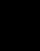Calculate how many words of Morse code to create compiling commas and dots in the words of one to four characters.
3. Subsets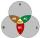How many are all subsets of set ??
4. Events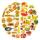Event N has probability of 0.24. What is the probability that the event N occurs in 8, 5, 4 try.
5. Bits, bytes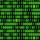Calculate how many different numbers can be encoded in 16-bit binary word?
6. Area codes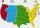How many 6 digit area codes are possible if the first number can't be zero?
7. Boys and girlsThere are eight boys and nine girls in the class. There were six children on the trip from this class. What is the probability that left a) only boys b) just two boys

We apologize, but in this category are not a lot of examples.
Do you have an interesting mathematical example that you can't solve it? Enter it, and we can try to solve it.

To this e-mail address, we will reply solution; solved examples are also published here. Please enter e-mail correctly and check whether you don't have a full mailbox.